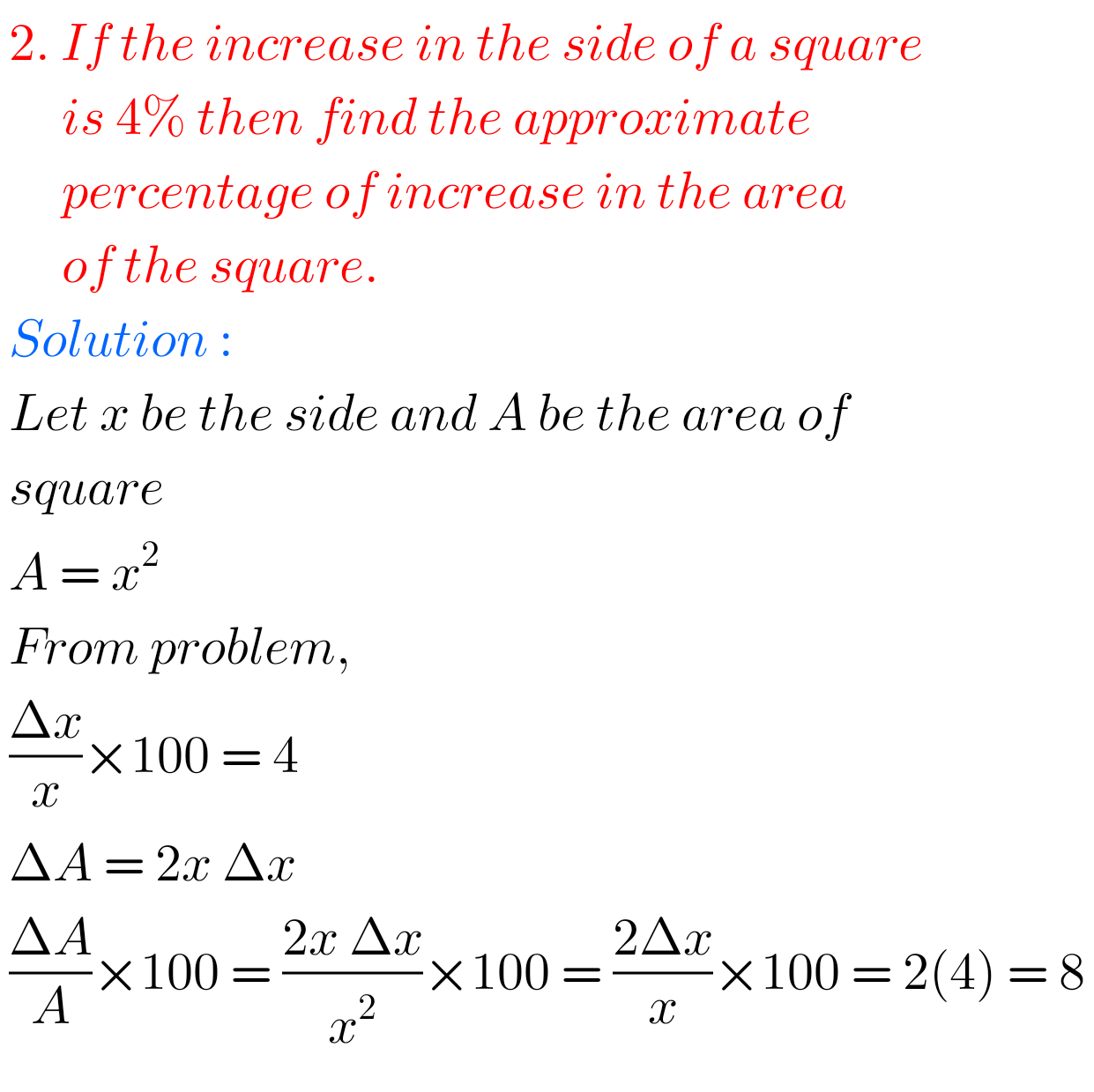## Applications of Derivatives Inter 1b solutions,chapter 10 Intermediate 1b solutions

Applications of derivatives inter 1b solutions  Mathematics 1B Intermediate Applications of Derivatives solutions are given.  These solutions are very simple to understand. Study the textbook lesson Applications of Derivatives very well. Intermediate mathematics first year 1b chapter 10 solutions You can also see the solutions  SSC Maths text book Solutions class 10 Inter maths 1A textbook …